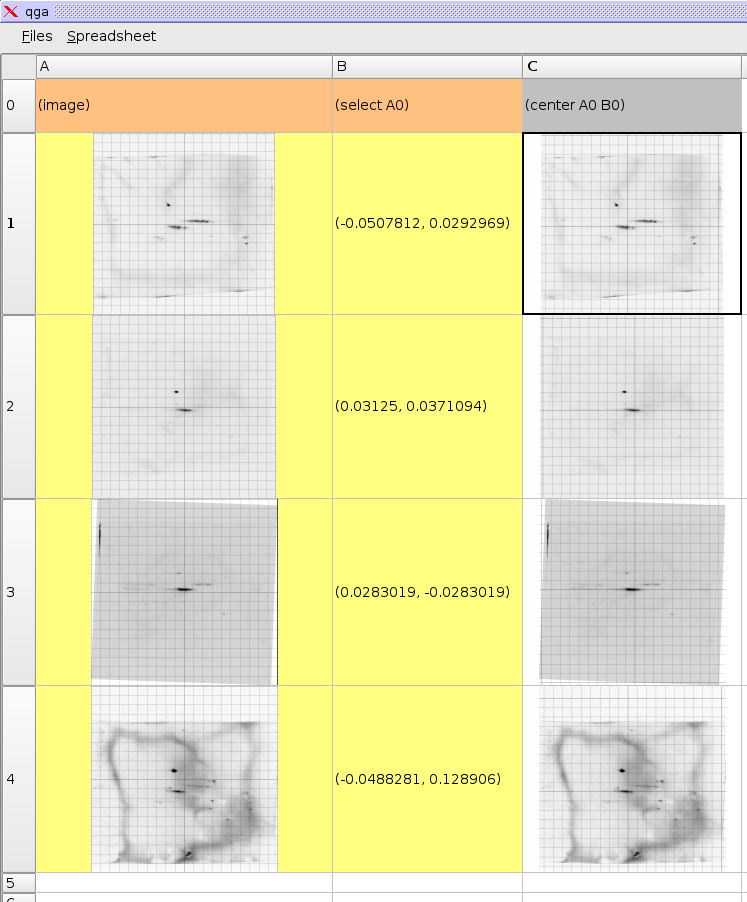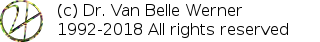Project Home

#Step 2: Centering 2DE Images

Explains how to assign the center of an image

`(select A0)(center A0 B0)`

When the images are loaded the spreadsheet assumes pixels width equal width as height. The orthonormal coordinate system reflects this and is painted on top of the pictures. At the moment these are clearly wrong. The first we need to do to resolve this is to define a center in all images. We do this by adding a new column with formulas.

Enter in cell B0 the formula (select A0) and in cell C0 the formula (center A0 B0). Copy these two down over the range B0:C2. The select operation requires user input and is thus painted orange. The operation will present the user with the image from cell A0 and ask to point to some feature. The selected coordinate will then become the value of that formula until a new position is entered. The center operation will shift the coordinate system such that the selected coordinate becomes the new center of the image.

 http://werner.yellowcouch.org/mailto:werner@yellowcouch.org